ProportionCustom SearchPROPORTION Closely allied with the study of ratio is the subject of proportion. A PROPORTION is nothing more than an equation in which the members are ratios. In other words when two ratios are set equal to each other, a proportion is formed. The proportion may be written in three different ways as in the following examples: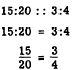The last two forms are the most common. All these forms are read, "15 is to 20 as 3 is to 4." In other words, 15 has the same ratio to 20 as 3 has to 4. One reason for the extreme importance of proportions is that if any three of the terms are given, the fourth may be found by solving a simple equation. In science many chemical and physical relations are expressed as proportions. Consequently, a familiarity with proportions will provide one method for solving many applied problems. It is evident from the last form shown,, that a proportion is really a fractional equation. Therefore, all the rules for fraction equations apply. TERMS OF A PROPORTION Certain names have been given to the terms of the two ratios that make up a proportion. In a proportion such as 3:8 = 9:24, the first and the last terms (the outside terms) are called the EXTREMES. In other words, the numerator of the first ratio and the denominator of the second are called the extremes. The second and third terms (the inside terms) are called the MEANS. The means are the denominator of the first ratio and the numerator of the second. In the example just given, the extremes are 3 and 24; the means are 8 and 9. Four numbers, such as 5, 8, 15, and 24, form a proportion if the ratio of the first two in the order named equals the ratio of the second two. When these numbers are set up as ratios with the equality sign between them, the members will reduce to an identity if a true proportion exists. For example, consider the following proportion: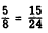In this proportion,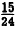must reduce tofor the proportion to be true. Removing the same factor from both members ofwe haveThe number 3 is the common factor that must be removed from both the numerator and the denominator of one fraction in order to show that the expression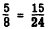is a true proportion. To say this another way, it is the factor by which both terms of the ratiomust be multiplied in order to show that this ratio is the same asPractice problems. For each of the following proportions, write the means, the extremes, and the factor of proportionality.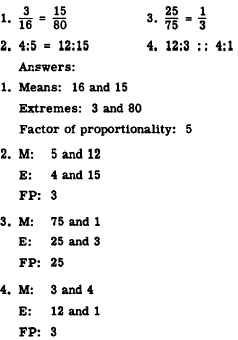Integrated Publishing, Inc. - A (SDVOSB) Service Disabled Veteran Owned Small Business